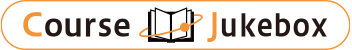### CourseDetailDegree
Bachelor
1
Course delivery methods
face-to-face
Subject
Physical sciences, Mathematical sciences, Engineering & technology
Program
School
School of Science and Engineering
Department
Bachelor's Program in Interdisciplinary Engineering
Campus
Tsukuba Campus
Classroom
3A405
Course Offering Year
2023-2024
Course Offering Month
November - February
Weekday and Period
Tue1,2
Capacity
Credits
2.0
Language
English
Course Number
FJ20134

### Introduction to Single-Variable Calculus II University of Tsukuba

#### Course Overview

This course along with "Introduction to Single- Variable Calculus I" and “Advanced Calculus” introduces the basic tools of calculus and develops their technical competence. The primary goal of this course is to understand the concepts and to build up a working ability of various mathematical manipulations such as parametric equations, polar coordinates, infinite sequences and series. This is efficiently achieved by visualization, numerical and graphical experimentations and students are required to be acquainted with Mathematica (or similar ones) during the course for working exercises and homework problems. The present course provides a basic core and practical knowledge required for many courses in both natural and social sciences.

#### Learning Achievement

1. The students are able to evaluate areas and volumes of two- and three-dimensional objects having revolution symmetry.
2. The students are able to calculate arc lengths by using parametric equations.
3. The students are able to understand and describe the conic sections with the cartesian and polar coordinates.
4. The students are able to understand the convergence of infinite sequences and series and evaluate those limits.

#### Competence

Related to 1. Mathematical logic and calculation skills.

#### Course prerequisites

* The students have taken "Introduction to Single- Variable Calculus I" or a similar level of Math courses.
* "Mathematica" is used to solve numerical problems. You are requested to install "Mathematica" in your own PC by the first lecture.
* Those who already earned the credit of Calculus I (FJ20104) are not allowed to earn the credit of this course.

Homework 30 %
Final 70%

#### Course schedule

Techniques of Integrals
Further Appl. Integrals
Exercises
Parametric Equations 1
Parametric Equations 2
Parametric Equations 3
Sequences and Series 1
Sequences and Series 2
Sequences and Series 3
Exercises and Summary

#### Course type

Lectures and Class Exercises

WANG JUNHAO

#### Other information

* You are strongly requested to work on many exercise problems in the textbook assigned during the lectures.
* All exams are closed book for in-class lecture. In the case of hybrid lecture, all exams are open book.

#### Site for Inquiry

Link to the syllabus provided by the university